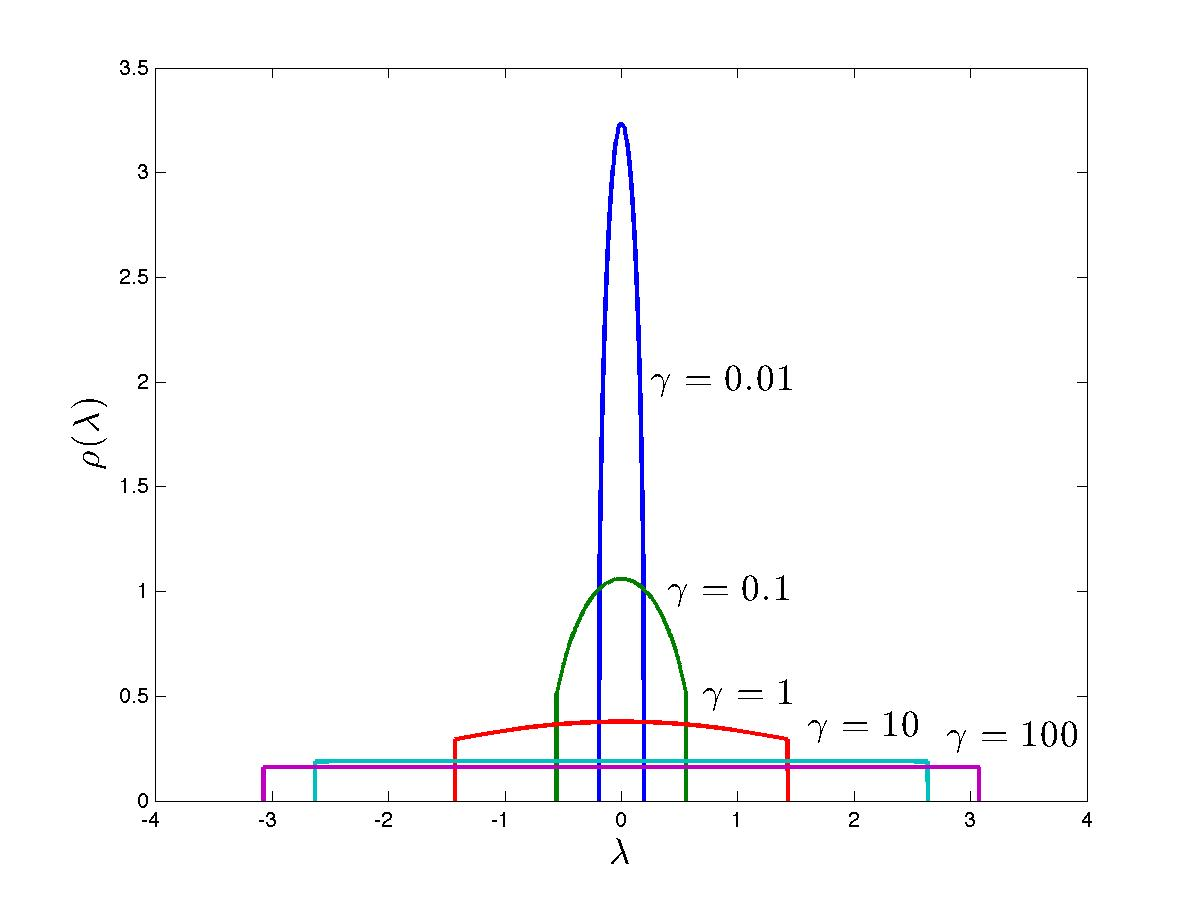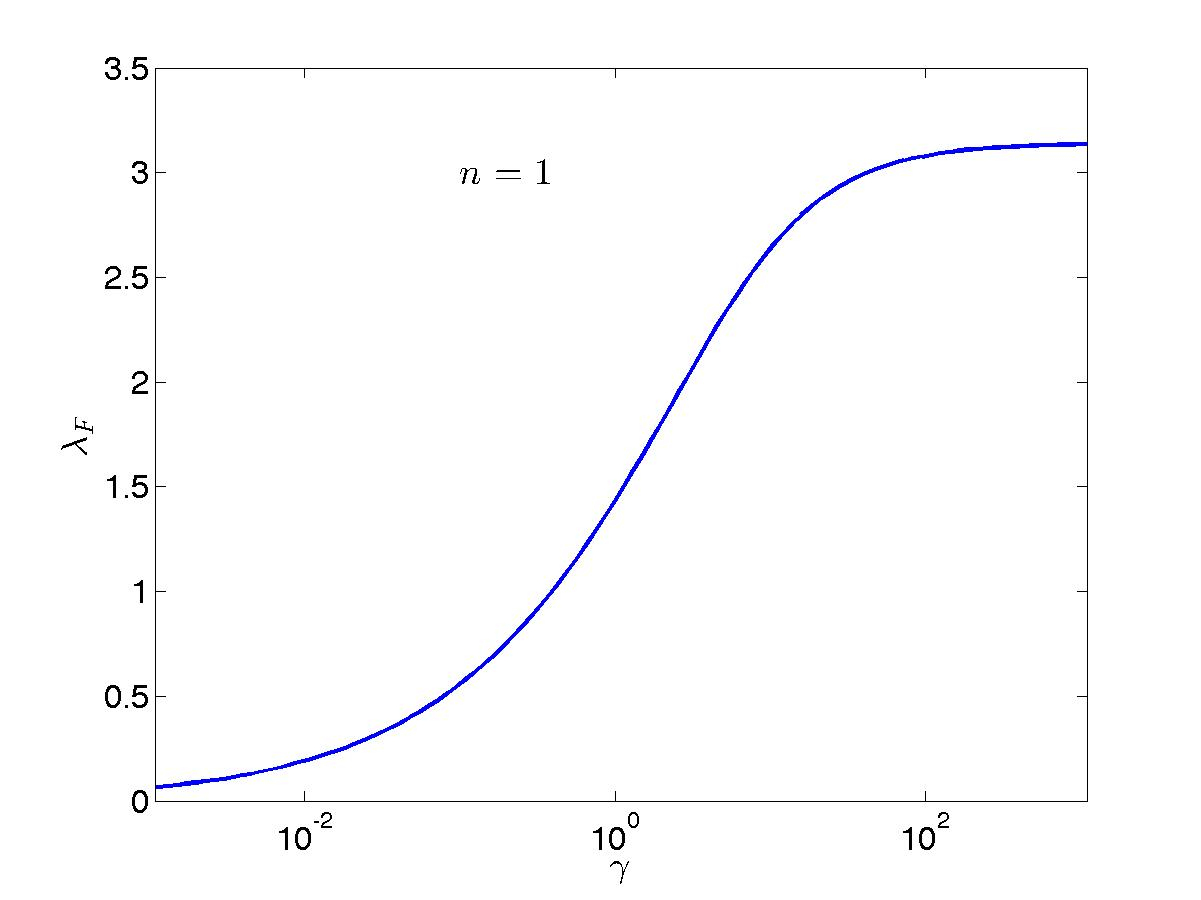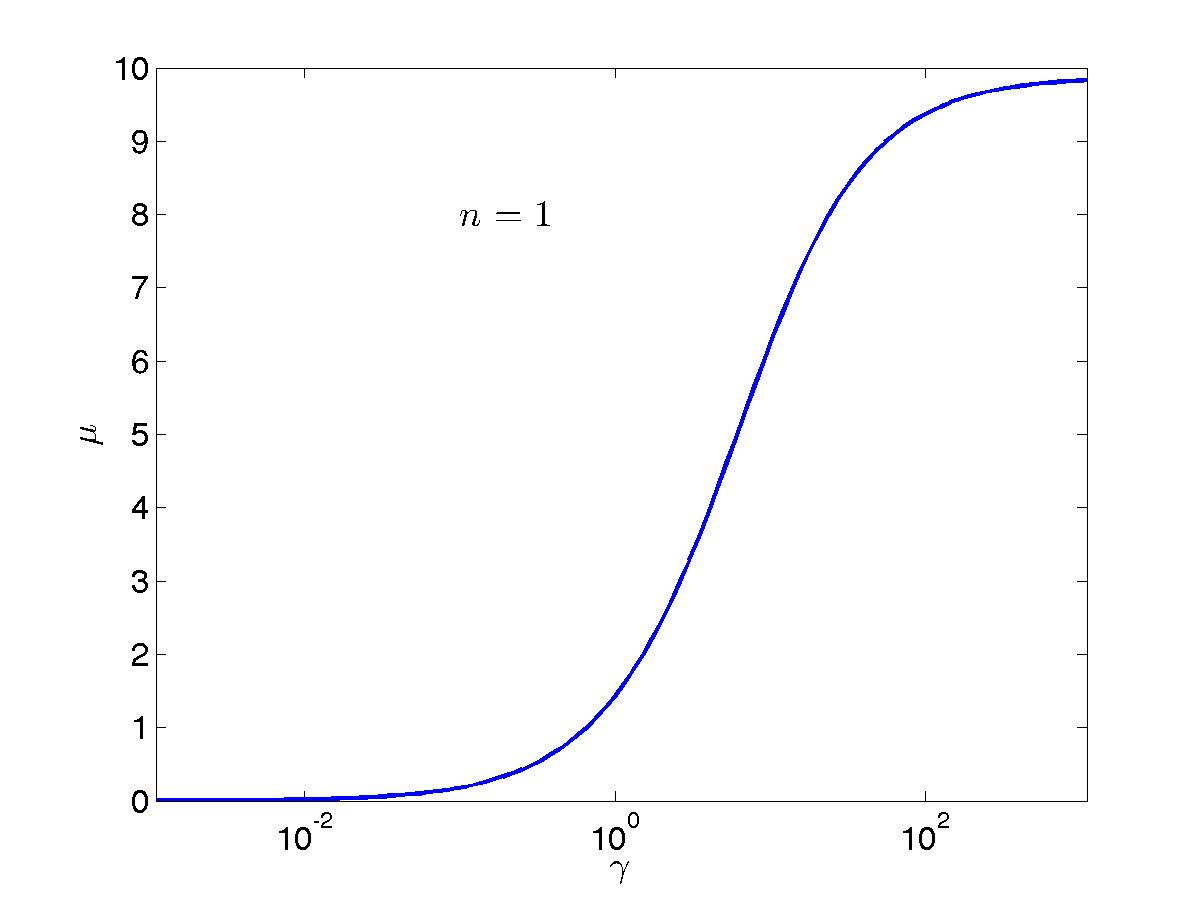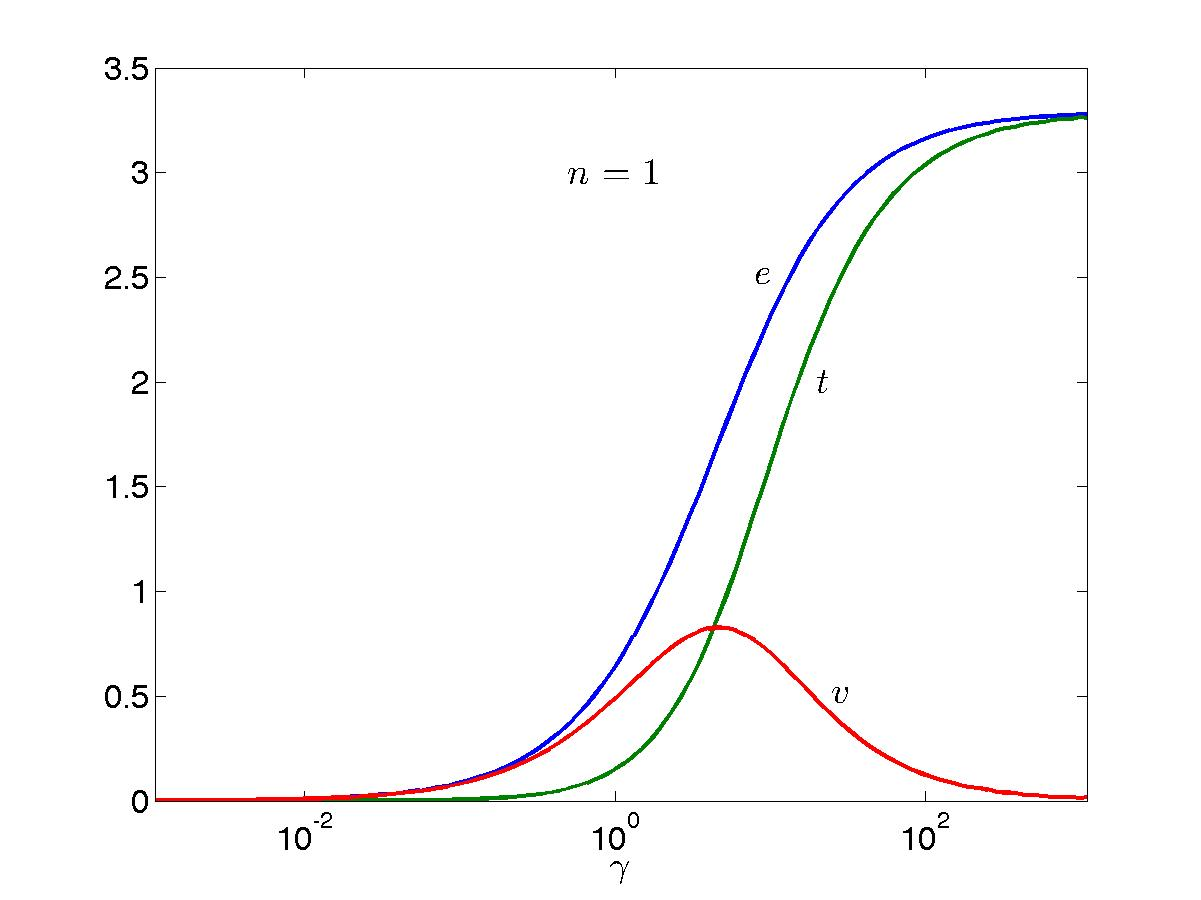# The Bethe Ansatz

#### The ground state and the Lieb equationg.l.Le

The ground state is obtained through choosing the following set of quantum numbers:

\begin{equation} I_j = -\frac{N+1}{2} + j, \hspace{1cm} j = 1, ..., N. \tag{l.igs}\label{l.igs} \end{equation}

In the thermodynamic limit, we can immediately obtain an integral equation for the corresponding ground state rapidity density function. We shall look for a ground state with finite particle density $$n$$. It is then natural to look for a density $$\rho_g(\lambda)$$ which is nonzero only within a finite interval $$\lambda \in [-\lambda_F, \lambda_F]$$ such that

\begin{align} \rho_g(\lambda) &= \left\{ \begin{array}{cc}\rho_t (\lambda), & -\lambda_F \leq \lambda \leq \lambda_F, \\ 0 & \mbox{otherwise} \end{array} \right. \nonumber \\ \rho_{g,h} (\lambda) &= \left\{ \begin{array}{cc}\rho_t (\lambda), & |\lambda| > \lambda_F, \\ 0 & \mbox{otherwise} \end{array} \right.. \tag{l.rg}\label{l.rg} \end{align}

The integral equation l.bec therefore becomes

\begin{equation} \rho_g(\lambda) - \int_{-\lambda_F}^{\lambda_F} d\lambda' {\cal C} (\lambda - \lambda') \rho_g(\lambda') = \frac{1}{2\pi}, \hspace{10mm} \lambda \in [-\lambda_F, \lambda_F] \tag{l.le}\label{l.le} \end{equation}

which is known as the Lieb equation. The Fermi momentum $$\lambda_F$$ can be viewed as an independent variable which (indirectly) specifies the particle density $$n$$, the relationship between these being

\begin{equation} n = \int_{-\lambda_F}^{\lambda_F} d\lambda ~\rho(\lambda). \tag{l.ng}\label{l.ng} \end{equation}

The density is a monotonically increasing function of $$\lambda_F$$ for $$c>0$$ (this will be proven later on), but the exact relationship cannot be written in closed form. In practice, one thus needs to solve l.le and l.ng self-consistently if one requires a specific particle density.

The Lieb equation is in fact identical to an equation known as Love's equation, occurring in classical electrostatics, and describing the capacitance of a circular capacitor. It does not admit a closed-form solution for $$0 < c < \infty$$. Expansions for small or large interactions can however be written down. See b-Gaudin for details.

Plots of the ground-state rapidity density function $$\rho(\lambda)$$ for a number of values of the interaction parameter can be found in Fig. FigLLgs. There, $$k_F$$ as a function of $$c$$ for unit filling $$n=1$$ is also plotted, together with the chemical potential $$\mu$$, as well as the total ($$e$$), kinetic ($$t$$) and potential ($$v$$) energy per unit lenght (in other words per particle at unit filling). These are simply calculated as

\begin{equation*} e = t + v, \hspace{10mm} v = c \frac{\partial e}{\partial c}. \end{equation*}

It is interesting to define the `maximally complicated' value of interaction for Lieb-Liniger as the point at which the kinetic energy density equals the interaction energy density (there is then no obvious starting point for any perturbative expansion). This occurs when $$t = v \simeq 0.82668(1)$$ at $$\gamma \simeq 4.3485(1)$$. Note that the interaction energy density has a maximum of $$\simeq 0.8277(1)$$ at a slightly higher value of the interaction $$\gamma \simeq 4.6572(4)$$, the kinetic energy density then being $$\simeq 0.8824(2)$$.The solution of the Lieb equation is particularly simple in the limit $$c \rightarrow \infty$$, since the Cauchy kernel vanishes. One then gets

\begin{equation*} \rho(\lambda)|_{c \rightarrow \infty} = \frac{\theta (\lambda_F - |\lambda|)}{2\pi}, \hspace{10mm} \lambda_F = \pi n. \end{equation*}

The Bethe equations l.bec then give the very simple hole density $$\rho_h$$ and total density $$\rho_t$$

\begin{equation*} \rho_h (\lambda)|_{c \rightarrow \infty} = \frac{\theta (|\lambda| - \lambda_F)}{2\pi}, \hspace{10mm} \rho_t (\lambda)|_{c \rightarrow \infty} = \frac{1}{2\pi}. \end{equation*}Except where otherwise noted, all content is licensed under a Creative Commons Attribution 4.0 International License.

Created: 2023-06-07 Wed 16:02